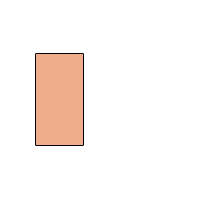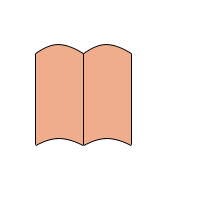Tutorials

How to design a simple note book

In this example you will learn to make simple note book, It is a very simple so follow this tutorial.

In this example you will learn to make simple note book, It is a very simple so follow this tutorial.

How to design a simple note book

In this example you will learn to make simple note book, It is a very simple so follow this tutorial.

New File: Create a new file with appropriate size.

Rectangle Shape: Choose Rectangle tool (U key) and make a rectangle shape with this "ECAF8D" color as looking down.Modify Shape: Choose Direct selection tool (U key) and click on the shape, the points will highlight then convert this shape.Make Another: Make duplicate layer and set it right side as here.Pen Tool: Choose Pen tool (P key) and points to make this shape.Add Lines: Take a new Layer and choose Brush = 1 with "black" color, now make some lines as here.You will have similar.

Final Image